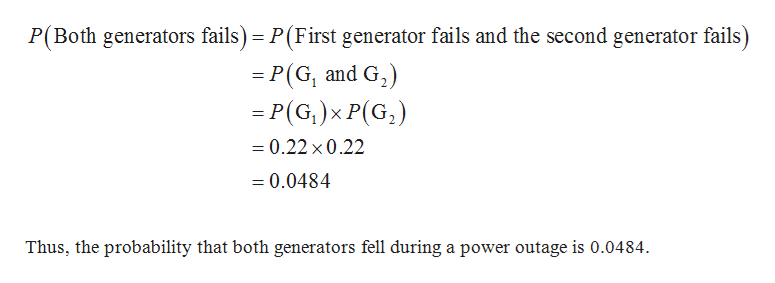Hospitals typically require backup generators to provide electricity in the event of a power outage assume the emergency back up generators feel 22% of the times when they are needed. The hospital has to back up generators so that the power is available if one of them fails during the power outage.  Find the probability to four decimal places that both generators fell during a power outage.

Question

Hospitals typically require backup generators to provide electricity in the event of a power outage assume the emergency back up generators feel 22% of the times when they are needed. The hospital has to back up generators so that the power is available if one of them fails during the power outage.  Find the probability to four decimal places that both generators fell during a power outage.

Step 1

Independent events:

If A and B are two independent events, the probability of the event A and B can be calculated as shown below:

Step 2

Computing the probability that both generators fell during a power outage:

It was found that the generator fails 22% of the times when they are needed.

There are two generators and the probability of each generator fell during a power outage is 0.22.

That is, the probability that the first generator, G1 fails is, P(G1) = 0.22.

Also, the probability that the second generator, G2 fails is, P(G2) = 0.22.

It is assumed that the ...help_outlineImage TranscriptioncloseP(Both generators fails) P(First generator fails and the second generator fails) -P(G, and G,) = P(G,)xP(G) =0.22 x 0.22 =0.0484 Thus, the probability that both generators fell during a power outage is 0.0484 fullscreen

Want to see the full answer?

See Solution

Want to see this answer and more?

Our solutions are written by experts, many with advanced degrees, and available 24/7

See Solution
Tagged in

Statistics